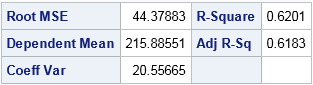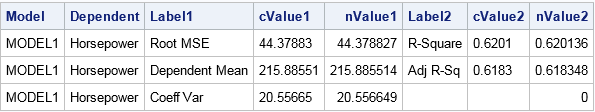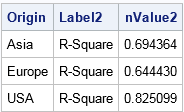In the beginning SAS created procedures and output. The output was formless and void. Then SAS said, "Let there be ODS," and there was ODS. Customers saw that ODS was good, and SAS separated the computation from the display and management of output.

The preceding paragraph oversimplifies the SAS Output Delivery System (ODS), but the truth is that ODS is a powerful feature of SAS. You can use ODS to send SAS tables and graphics to various output destinations, including HTML, PDF, RTF, and PowerPoint. You can control the style and attributes of the output, thus creating a customized report. There have been hundreds of papers and books written about ODS. A very basic introduction is Olinger (2000) "ODS for Dummies."

To a statistical programmer the most useful destination is the OUTPUT destination. The OUTPUT destination sends a table or graph to a SAS data set. Consequently, you can programmatically access each element of the output.

The implications of the previous statement are monumental. I cannot overstate the importance of the OUTPUT destination, so let me say it again:

The ODS OUTPUT destination enables you to store any value that is produced by any SAS procedure. You can then read that value by using a SAS program.

The ODS OUTPUT destination answers a common question that is asked by new programmers on SAS discussion forums: "How can I get a statistic into a data set or into a macro variable?" The steps are as follows:

1. Use ODS TRACE ON (or the SAS documentation) to find the name of the ODS table that contains the statistic that you want.
2. Use the ODS OUTPUT statement to specify the table name and a data set name. The syntax is ODS OUTPUT TableName=DataSetName. Then run the procedure to generate the table.
3. Read the data set to obtain the value of the statistic.

New to #SAS programming? How to get any statistic into a data set.
Click To Tweet

### Find the name of the ODS table

As an example, suppose that you intend to use PROC REG to perform a linear regression, and you want to capture the R-square value in a SAS data set. The documentation for the procedure lists all ODS tables that the procedure can create, or you can use the ODS TRACE ON statement to display the table names that are produced by PROC REG. The data are the 428 vehicles in the Sashelp.Cars data set, which is distributed with SAS:

```ods trace on; /* write ODS table names to log */ proc reg data=Sashelp.Cars plots=none; model Horsepower = EngineSize Weight; quit; ods trace off; /* stop writing to log */``````Output Added: ------------- Name: FitStatistics Label: Fit Statistics Template: Stat.REG.FitStatistics Path: Reg.MODEL1.Fit.Horsepower.FitStatistics -------------```

By looking at the output, you can see that the third table contains the R-square value. By looking at the SAS log, you can see that the name of the third table is "FitStatistics."

### Save the table to a SAS data set

Now that you know the name of the ODS table is "FitStatistics," use the ODS OUTPUT destination to write that table to a SAS data set, as follows:

```ods output FitStatistics=Output; /* the data set name is 'Output' */ proc reg data=Sashelp.Cars plots=none; /* same procedure call */ model Horsepower = EngineSize Weight; quit;   proc print data=Output noobs; run;```The output from PROC PRINT shows the structure of the output data set. Notice that the data set often looks different from the original displayed table. The data set contains non-printing columns (like Model and Dependent) that do not appear in the displayed table. The data set also contains columns that contain the raw numerical values and the (formatted) character values of the statistics. The columns cValue1 and nValue1 represent the same information, except that the cValue1 is a character column whereas nValue1 is a numerical column. The same applies to the cValue2 and nValue2 columns. The character values might contain formatted or rounded values.

### Read the value of the statistic into a macro variable

From the previous PROC PRINT output, you can see that the numerical value of the R-square statistic is in the first row and is in the nValue2 column. You can therefore read and process that value by using a standard WHERE clause. For example, the following statements use the SYMPUTX subroutine to create a macro variable that contains the value of the R-square statistic:

```data _null_; set Output; if Label2="R-Square" then call symputx("RSq", nValue2); run;   %put RSq = &RSq;```
`RSq = 0.6201360929`

The SAS log shows that the R-square value is now contained in the Rsq macro variable.

Storing the statistic in a macro variable is only one way to use the data set. You could also read the statistics into PROC IML or PROC SQL for further computation, or show the value of the statistic in a graph.

### BY-group processing: Multiple samples and multiple statistics

The previous sections show how to save a single table to a SAS data set. It is just as easy to create a data set that contains multiple statistics, one for each level in a BY-group analysis.

Suppose that you want to run several regressions, one for each value of the Origin variable, which has the values "Asia," "Europe," and "USA." The following call to PROC SORT sorts the data by the Origin variable. The sorted data is stored in the CARS data set.

```proc sort data=Sashelp.Cars out=Cars; by Origin; run;```

You can then specify Origin on the BY statement in PROC REG to carry out three regression analyses. When you run a BY-group analysis, you might not want to see all of the results displayed on the computer screen, especially if your goal is to save the results in an output data set. You can use the ODS EXCLUDE statement to suppress SAS output.

```ods exclude all; /* suppress tables to screen */ ods output FitStatistics=Output2; /* 'Output2' contains results for each BY group */ proc reg data=Cars plots=none; by Origin; model Horsepower = EngineSize Weight; quit; ods exclude none; /* no longer suppress tables */   proc print data=Output2 noobs; where Label2="R-Square"; var Origin Label2 nValue2; run;```The output from PROC PRINT shows the R-square statistics for each model. Notice that the BY-group variables (in this case, Origin) are added to output data sets when you run a BY-group analysis. You can now use the statistics in programs or graphs.

### Alternatives to using ODS OUTPUT

Some procedures provide an alternative option for creating an output data set that contains statistics. Always check the SAS documentation to see if the procedure provides an option that writes common statistics to an output data set. For example, the documentation for the PROC REG statement states that you can use the OUTEST= option with the RSQUARE option to obtain an output data set that contains the parameter estimates and other model statistics such as the R-square value. Thus for this example, you do not need to use the ODS OUTPUT statement to direct the FitStatistics table to a data set. Instead, you can obtain the statistic as follows:

```proc reg data=Cars NOPRINT outest=Output3 RSQUARE; /* statistics in 'Output3' */ by Origin; model Horsepower = EngineSize Weight; quit;   proc print data=Output3 noobs; format _RSQ_ 8.6; var Origin _RSQ_; run;```### Summary

In summary, the ODS OUTPUT statement enables you to create a data set that contains any statistic that is produced by a SAS procedure. You can use the ODS OUTPUT statement to capture a statistic and use it later in your program.

tags: Getting Started

The post ODS OUTPUT: Store any statistic created by any SAS procedure appeared first on The DO Loop.